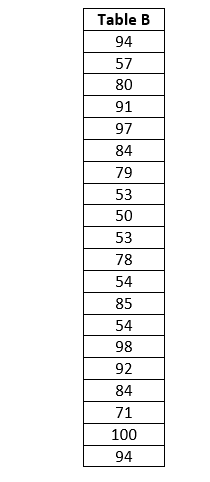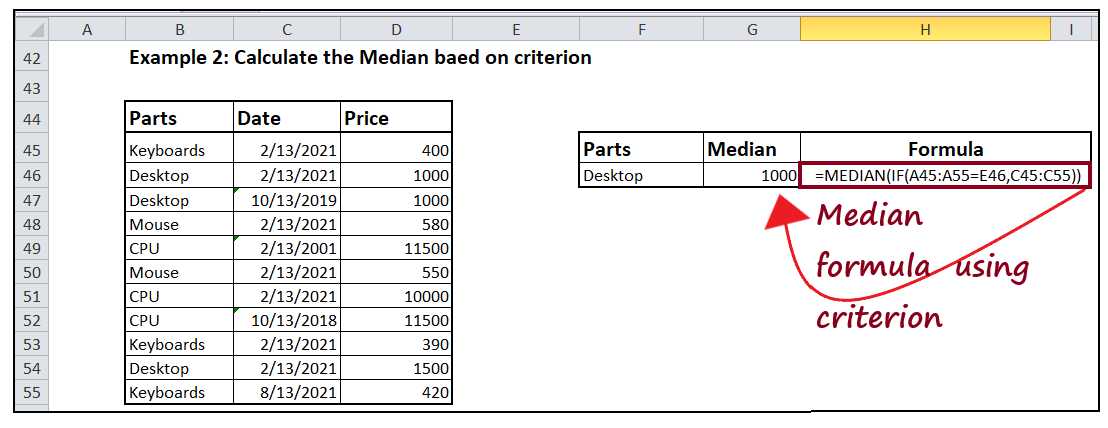# Excel Median() function

In simple mathematical terms, the median is defined as the middle value in a given data set, separating the higher half of data values from the lower half. More specifically, it is the middle value of the data set where the elements are arranged in order of magnitude.

Similarly, “The Excel Median() function returns a number which is in the center value of all numbers in the given data set“.

The formula to calculate the median is different for even and an odd group of numbers. For example, in a data set containing an odd number of values, the median will be the middle value of all numbers. In contrast, for a data set containing an even group of numbers, the median is fetched by calculating the average of the middle two numbers.#### NOTE: Median is calculated after arranging the data set either in ascending or descending order. In Excel, the orderly arrangement of the data set won’t be visible as it is done in the backend.

People always confuse mean with median and sometimes use the words interchangeably. But both are different as they mean the average value of a group of numbers. In contrast, the median is the middle value when the data set is arranged in ascending or descending order. The median is less susceptible to outliers (extremely large or small data values). Therefore, it is advised to use MEDIAN in calculations for asymmetrical distribution. A perfect example is a median salary as it gives a better idea of how much the employees earn in particular. In contrast, if you have calculated the mean salary, there are chances the figures may get twisted due to extremely low or high salary figures.

### Syntax

=MEDIAN([number1],[number2], and so on..)

### Parameters

1. number1 (required): This parameter represents the first number of the list. It is mandatory to enter at least one number in the median function.
2. [number2], and so on.. (optional) : This parameter represents the list of numbers for which you want to find the median. These can hold any values, including numbers, dates (where dates are mentioned in a common format), named ranges, arrays, or even cell references.

## 4 Facts to remember about Excel Median

1. When the data set contains an odd number of values, the median will be the middle value of all numbers. In contrast, for a data set containing an even group of numbers, the median is fetched by calculating the average of the middle two numbers.
2. The Median() function also includes the cells with zero values (0) in the calculations.
3. Though the Median() function does not include empty cells and the cells that contain text and logical values.
4. The Median() function counts Boolean values in the data set. If the user passes Boolean FALSE in the parameters, it reads it as 0, and if the user passes Boolean TRUE, it reads it as 1. For instance, the formula MEDIAN (1, 4, TRUE, FALSE, 3) returns 0, which is the median of the data set {1, 4, 0, 1, 3}.

### Examples

Example 1: Calculate median for the following numbers.

a) Table ASolution: Select a cell and type the below formula to calculate the median of the above table.

Formula Used: =MEDIAN(A15:A35)If you sort the values ascending, and count the number of observations here, we have 21 (in Table A) and the middle observation would be the 11th observation which has a value of 84.

b) Table BSolution: Select a cell and type the below formula to calculate the median of the above table.

Formula Used: =MEDIAN(G15:G35)If the number of observations would have been 20 (as is the case in Table B), then the median would have been 10th + 11th observation divided by 2 which will be our median.

As demonstrated in the above example, the Excel Median formula works in the same manner for number and date values because dates are considered number values in Excel. Go ahead and try the MEDIAN formula for Dates values as well!

Example 2: Find out the median of the below table based on criterion. Calculate median where the Parts are equal to Desktop.Although there are no special functions in Microsoft Excel to calculate a median based on conditions, as we know, Excel allows us to create a customised formula by merging two or more functions. The same trick we can use to build our own MEDIAN formula integrating it with the IF function.

Formula Used

=MEDIAN(IF(table_range=criteria, median_range))

Explanation of Formula:

To find the median, we have used the MEDIAN formula. Inside the MEDIAN formula, we have incorporated the IF function. Inside IF, we will check whether the table range is equal to the criteria value (Range (A45: A55) = Desktop) or not. If the ‘IF’ function returns true, it will return the median_range (D45: D55). After that, the Median function will come into play to find the median for the returned range.In the above screenshot, to find a median amount for Desktop, we have created another table with parts name, median and formula and with the help of following formula to we fetched the median of the specific item based on that condition:

=MEDIAN(IF(B45:B55=F46,D45:D55))

In the above example, we have found the Median based on single criteria, but you can also indulge more and can use the Median formula for multiple criterions. Go ahead and give it a try!

Next Topic#